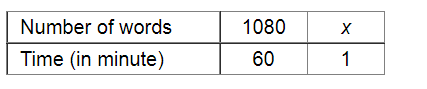# Suneeta types 1080 words in one hour.`
Question:

Suneeta types 1080 words in one hour. What is her GWAM (gross words a minute rate)?

Solution:Let $x$ be her GWAM.

If the time taken is less, GWAM will also be less.

Therefore, it is a direct variation.

$1080: x=60: 1$

$\Rightarrow \frac{1080}{x}=\frac{60}{1}$

Applying cross muliplication, we get:

$x=\frac{1080 \times 1}{60}$

$=18$

Thus, her GWAM will be 18 .